Connect with us

# Mix and Yield Variance AnalysisPublished

onVariance analysis is a most used tool by accountants to review and control of responsibility center operations [that use standard cost system] and as facilitating factors in judging managerial performance in general. I have discussed single variance analyses on various post in the past, but I haven’t discussed mix and yield variance analysis yet. So, in this post I discuss mix and yeild variance analysis. Through a single case example demonstrated in this post, you will learn what mix variance is, what yield variance is and how both types of variance computed and recorded. Enjoy!

### What Are Mix And Yield Variances?

The material mix variance measures the impact of the deviation from the standard mix on material costs. The material yield variance measures the impact on material costs of the deviation from the standard input material allowed for actual production.

### What Causes Mix And Yield Variances?

Possible causes of unfavorable mix variances are:

• When capacity restraints force substitution
• Poor production scheduling
• Lack of certain types of labor
• Short supply of certain materials

Possible causes of unfavorable yield variances are:

• Low quality materials and/or labor
• Faulty equipment
• Improper production methods
• Improper or costly mix of materials and/or labor

### How To Compute Mix And Yield Variances?

• The material quantity variance is divided into a material mix variance and a material yield variance.
• Compute the material mix variance by holding the total input units constant at their actual amount.
• Compute the material yield variance by holding the mix constant at the standard amount.
• The computations for labor mix and yield variances are the same as those for materials. If there is no mix, the yield variance is the same as the quantity (or usage) variance.

### Mix and Yield Variance Analysis Case Example

A company uses a standard cost system for its production of a chemical product. This chemical is produced by mixing three major raw materials, A, B, and C. The company has the following standards:

36 lb of Material A @1.00           = \$36.00
48 lb of Material B @2.00            = \$96.00
36 lb of Material C @1.75            = \$63.00
120 lb of standard mix @1.625 = \$195.00

The company should produce 100 lb of finished product at a standard cost of \$1.625 per lb (\$195/120 lb). To convert 120 lb of materials into 100 lb of finished chemical requires 400 direct labor hours at \$3.50 per hour, or \$14 per lb. During the month of December, the company completed 4,250 lb of output with the following labor: direct labor 15,250 hours @\$3.50. Material records show: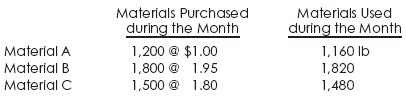Note: The material price variance is isolated at the time of purchase.

We want to compute the material purchase price, quantity, mix, and yield variances. We also want to prepare appropriate journal entries.

I will show the computations of variances in a tabular form as follows:

### The Material Variance Analysis

Material Purchase Price Variance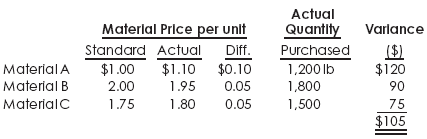The material quantity variance computed below results from the change in the mix of materials as well as from changes in the total quantity of materials. The standard input allowed for actual production consists of 1,275 lb of Material A, 1,700 lb of Material B, and 1,275 lb of Material C, a total of 4,250 lb. The actual input consisted of 1,160 lb of Material A, 1,820 lb of Material B, and 1,480 lb of Material C.

The total of variance is subdivided into a material mix variance and a material yield variance, as shown below.

### Material Quantity Variance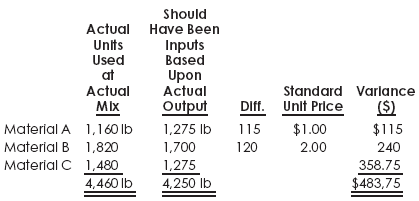The computation of the material mix variance and the material yield variance for the Giffen Manufacturing Company is given below:

### Material Mix Variance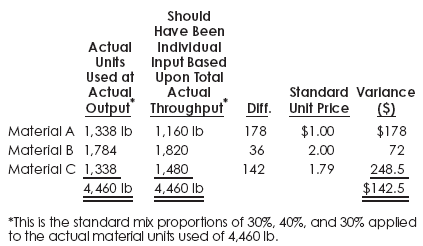### Material Yield Variance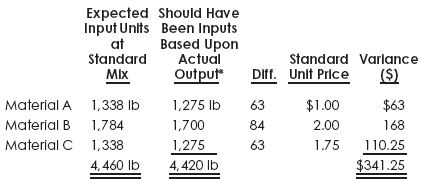Notes:

*This is the standard mix proportions of 30%, 40%, and 30% applied to the actual throughput of 4,460 lb or output of 4,250 lb.

**The material yield variance of \$341.25 U can be computed alternatively as follows.

Actual input quantity at standard prices:

Material A 1,338 lb @ \$1.00 = \$1,338.00
Material B 1,784 lb @ 2.00   = \$3,568.00
Material C 1,338 lb @ 1.75  =  \$2,341.50
Total                                    = \$7,247.50

Hence, \$7,247.5 ? \$4,906.25= \$341.25

The material mix and material yield variances are unfavorable, indicating that a shift was made to a more expensive (at standard) input mix and that an excessive quantity of material was used. Poor production scheduling requiring an unnecessarily excessive use of input material and an undesirable mix of Material A, B, and C was responsible for this result. To remedy the situation, the company must ensure that:

• The material mix is adhered to in terms of the least cost combination without affecting product quality;
• The proper production methods are being implemented; Inefficiencies, waste, and spoilage are within the standard allowance; and
• Quality materials, consistent with established standards are being used.

### Journal Entries For Material Mix and Yield Variance

Here are journal entries involved:

. Journal Entry To Record Material Purchases:

[Debit]. Material and Supplies = 7,425*
[Debit]. Material Purchase Price Variance = 105
[Credit]. Cash (or Accounts Payable) = 7,530**

Notes:

*Actual quantities purchased at standard prices:

Material A (1,200 lb @ \$1.00) = \$1,200
Material B (1,800 @ 2.00)       = \$3,600
Material C (1,500 @ 1.75)       = \$2,625
Total =                                   = \$7,425

**Actual quantities purchased at actual prices:

Material A (1,200 lb @ \$1.10) = \$1,320
Material B (1,800 @ 1.95)       = \$3,510
Material C (1,500 @ 1.80)       = \$2,700
Total                                      = \$7,530

. Journal Entry To Charge Materials into Production:

[Debit]. Work-in-Process = \$7,247.50*
[Debit]. Material Mix Variance = 42.50
[Credit]. Material and Supplies = 7,390**

Notes:

* Actual quantities used at standard mix at standard prices:

Material A (1,338 lb @ \$1.00) = \$1,338.00
Material B (1,784 @ 2.00)        = \$3,568.00
Material C (1,480 @ 1.75)        = \$2,341.50
Total                                        = \$7,247.50

** Actual quantities used at standard prices:

Material A (1,160 lb @ \$1.00) = \$1,160.00
Material B (1,820 @ 2.00)        = \$3,640.00
Material C (1,480 @ 1.75)       = \$2,590.00
Total                                       = \$7,390.00

. Journal Entry To Transfer Material Costs to Finished Goods

[Debit]. Finished Goods = \$6,906.25*
[Debit]. Material Yield Variance = 341.25
[Credit]. Work-in-Process = 7,240.50*

Note:

*See the previous table for the numerical computations.

### The Labor Variance Analysis

Employees seldom complete their operations according to standard times. Two factors should be considered in computing labor variances if the analysis and computation are to be used to fix responsibility:

• The change in labor cost resulting from the efficiency of the workers, measured by a labor efficiency variance. (In finding the change, allowed hours are determined through the material input).
• The change in labor cost due to a difference in the yield, measured by a labor yield variance. (In computing the change, actual output is converted to allowed input hours).

For the Manufacturing Company, more efficient workers resulted in a savings of 383.33 hours (15,250 hr – 14,866.67 hr). Priced at the standard rate per hour, this produced an unfavorable labor efficiency variance of \$1,341.66 as shown below:
Labor Efficiency Variance

Actual hrs. at standard rate                            =  \$53,375.00
Actual hrs. at expected output                       = (\$52,033.30)
(4,460 hr x 400/120) = 14,866.67 hr @ \$3.5 = \$ 1,341.60

With a standard yield of 83 1/3% (=100/120), 4,250 lb of finished material should have required 17,000 hr of direct labor (4,250 lb x 400 DLH/100). Comparing the hours allowed for the actual input 14,866.67 hr with the hours allowed for actual output, 17,000 hr, we find a favorable labor yield variance of \$7,466.66, as shown below:

Labor Yield Variance

Actual hr at expected output                        = \$52,033.30
Actual output (4,250 lb x 400/100
= 17,000 hrs. @ \$3.5 or 4,250 lb @ \$14.00) = \$59,500.00
Labor Yield Variance                                     = \$ 7,466.60

Labor Efficiency Variance

The labor efficiency variance can be combined with the yield variance to give us the traditional labor efficiency variance, which turns out to be favorable as follows:

Labor efficiency variance   = \$1,341.66
Labor yield variance           = \$7,466.66
Labor Effeciency Variance  = \$6,125.00

This division is necessary when there is a difference between the actual yield and standard yield, if responsibility is to be fixed. The producing department cannot be rightfully credited with a favorable efficiency variance of \$6,125. Note, however, that a favorable yield variance, which is a factor most likely outside the control of the producing department, more than offsets the unfavorable labor efficiency variance of \$1,341.66, for which the producing department rightfully should have been responsible.

### Journal Entries For Labor Mix and Yield Variance

. To Transfer Labor Costs to Work-in-Process:

[Debit]. Work-in-Process = 52,033.34
[Debit]. Labor Efficiency Variance = 1,341.66
[Credit]. Payroll = 53,375.00

. To Transfer Labor Costs to Finished Goods:

[Debit]. Finished Goods = 59,500.00
[Credit]. Labor Yield Variance = 7,466.66
[Credit]. Work-in-Process = 52,033.34

Are you looking for easy accounting tutorial? Established since 2007, Accounting-Financial-Tax.com hosts more than 1300 articles (still growing), and has helped millions accounting student, teacher, junior accountants and small business owners, worldwide.